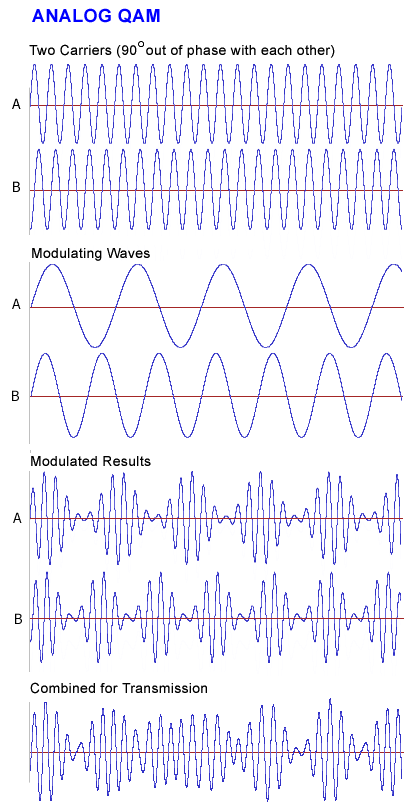# Definition: QAM

(1) (Quality Assessment Measurement) A system used to measure and analyze voice transmission.

(2) (Quadrature Amplitude Modulation) A modulation technique that employs both phase modulation (PM) and amplitude modulation (AM). Widely used to transmit digital signals such as digital cable TV and cable Internet service, QAM is also used as the modulation technique in orthogonal frequency division multiplexing (see OFDM). The "quadrature" comes from the fact that the phase modulation states are 90 degrees apart from each other.

Analog QAM
Analog QAM uses two carriers 90 degrees out of phase with each other. Each carrier is modulated by an analog signal, and the resulting modulated waves are combined (see example below).

Digital QAM
In digital QAM, the number of modulation states determines how the digital signal is split up. For example, in 8QAM, each three bits of input alters the phase and amplitude of the carrier to derive eight unique modulation states (see example below).

In 16QAM, 32QAM, 64QAM, 128QAM, 256QAM, 512QAM and 1024QAM, from four to 10 bits generate from 16 to 1,024 modulation states respectively. See modulation and binary values.Analog QAM
Analog QAM modulates two carriers 90 degrees out of phase with each from two analog input streams. The modulated carriers are combined and transmitted.Digital QAM (8QAM)
In 8QAM, three input bits generate eight modulation states using four phase angles on 90 degree boundaries and two amplitudes (4 phases X 2 amplitudes = 8 states). Digital modulation greater than 8QAM is difficult to visualize.Quadrature PSK (QPSK)
QPSK is like 4QAM without amplitude modulation. QPSK uses four phase angles to represent each two bits of input; however, the amplitude remains constant.